# 7. Let P be the plane given by r+y+s=3. Let q= (4,2,3). Find the point on P that is closest toq.

Question
8 views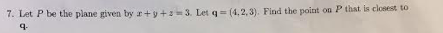help_outlineImage Transcriptionclose7. Let P be the plane given by r+y+s=3. Let q= (4,2,3). Find the point on P that is closest to q. fullscreen
check_circle

Step 1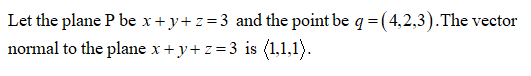Step 2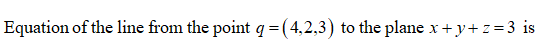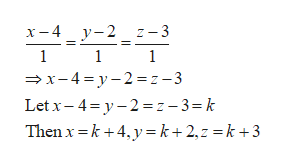help_outlineImage Transcriptioncloseх-4 _у-2 1— 3 — х-4%3Dу-2 %3D2-3 Let x- 4= y- 2 = z- 3= k Then x =k +4, y= k + 2,z = k +3 fullscreen
Step 3

Substitute the values of x, y, z in the plane to find the p...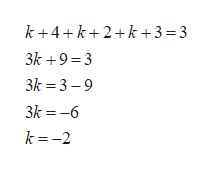help_outlineImage Transcriptionclosek +4+k+2+k +3=3 3k +9=3 3k = 3 – 9 3k = -6 k = -2 fullscreen

### Want to see the full answer?

See Solution

#### Want to see this answer and more?

Solutions are written by subject experts who are available 24/7. Questions are typically answered within 1 hour.*

See Solution
*Response times may vary by subject and question.
Tagged in

### Math